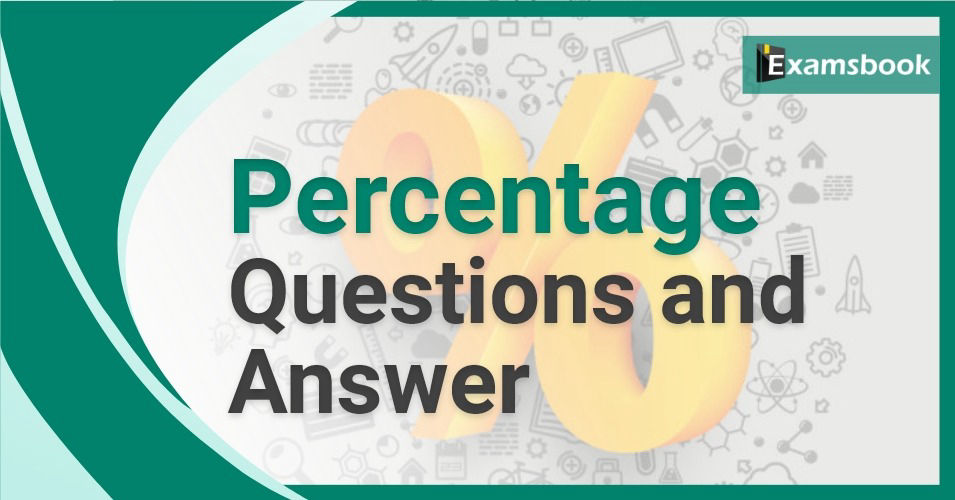• Save

# Percentage Questions and Answers of Aptitude for SSC and Bank ExamMost of the students face difficulties while solving percentage questions and answers of aptitude in competitive exams. In this post, you can practice with percentage aptitude questions and answers which questions asked mostly in competitive exams like SSC and banking exams.

You can score good marks in this topic by solving these 60 percentage aptitude questions with answers. Check percentage problems with solutions that how to solve percentage problems with different equations examples.

## Percentage Aptitude Questions and Answers

Q.1 What percent of 7.2 is 6?

(A) 10%

(B) 20%

(C) 8 1/3 %

(D) None

Ans .  C

To know more about Profit and Loss: profit and loss aptitude questions

Q.2 If 30% of a number is 12.6, then the number is …….

(A) 41

(B) 51

(C) 52

(D) 42

Ans .  D

Q.3 What will be 160% of a number whose 200% is 140?

(A) 200

(B) 160

(C) 140

(D) 112

Ans .  D

Q.4 If A’s income is 33 1/3 % more than that of B, then how much percent is B’s income less than that of A?

(A) 25%

(B) 33 1/3 %

(C) 40%

(D) None

Ans .  A

Q,5 63% of 3 4/7 is…….

(A)2.25

(B) 2.40

(C) 2.50

(D) 2.75

Ans .  A

Q.6 What percentage is 3% of 5%?

(A) 60%

(B) 50%

(C) 15%

(D) 30%

Ans .  A

Q.7 A number increased by 37 ½ % gives 33. The number is….

(A) 27

(B) 25

(C) 24

(D) 22

Ans .  C

Q.8 40 quintals is what percent of 2 m tones?

(A) 20 %

(B) 2%

(C) 200%

(D) 150%

Ans .  C

Q.9 If 15% of 40 is greater than 25% of a number by 2, then number is….

(A) 16

(B) 20

(C) 24

(D) 32

Ans .  A

Q.10 12.5% of 192=50% of?

(A) 48

(B) 96

(C) 24

(D) None

Ans .  A

If you have any query or want to ask anything regarding percentage questions and answers, you can ask me in the comment box. Visit on next page for more practice.